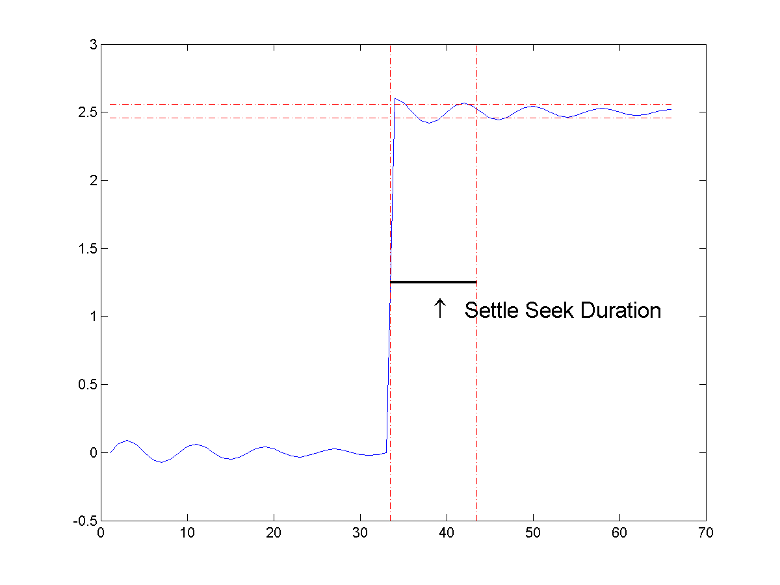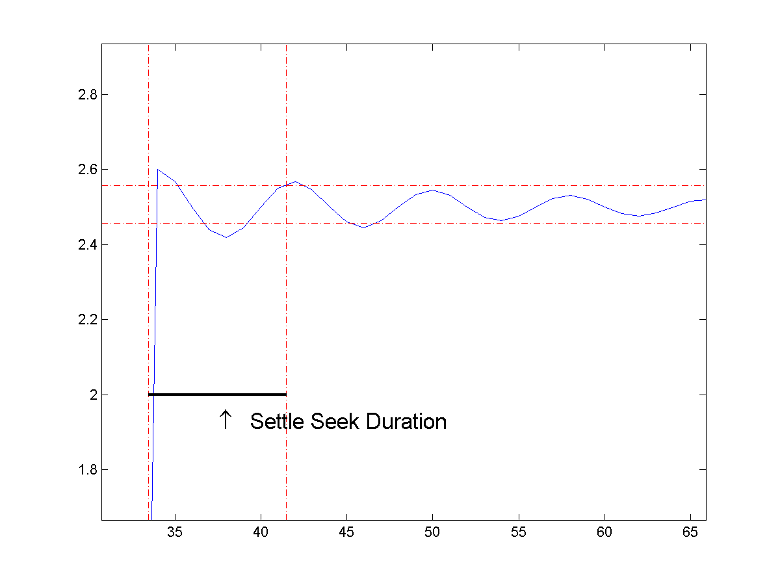# settlingtime

Settling time for bilevel waveform

## Syntax

s = settlingtime(x,d)
s = settlingtime(x,Fs,d)
s = settlingtime(x,t,d)
 [s,slev,sinst] = settlingtime(___)
 [s,slev,sinst] = settlingtime(___,Name,Value)
settlingtime(___)

## Description

example

s = settlingtime(x,d) returns the time from the mid-reference level instant to the time instant each transition enters and remains within a 2% tolerance region of the final state over the duration d. To determine the transitions, the settlingtime function estimates the state levels of the input waveform by a histogram method and identifies all regions that cross the upper-state boundary of the low state and the lower-state boundary of the high state. NoteIf for any transition, the level of the waveform does not remain within the lower and upper tolerance boundaries, the requested duration is not present, or an intervening transition is detected, settlingtime marks the corresponding element in s as NaN. For cases in which settlingtime returns a NaN, see Settle Seek Duration. 

example

s = settlingtime(x,Fs,d) specifies the sample rate Fs.
s = settlingtime(x,t,d) specifies the sample instants t.

example

 [s,slev,sinst] = settlingtime(___) returns the levels slev and sample instants sinst of the settling points for each transition.
 [s,slev,sinst] = settlingtime(___,Name,Value) returns the settling times, levels, and corresponding sample instants with additional options specified by one or more name-value arguments. You can specify an input combination from any of the previous syntaxes.

example

settlingtime(___) plots the signal and darkens the regions of each transition where settling time is computed. The plot marks the location of the settling time of each transition, the mid-crossings, and the associated reference levels. The plot also displays the state levels with the corresponding lower and upper tolerance boundaries. 

## Examples

collapse all

Determine the settling point and corresponding waveform value for a bilevel waveform. Specify a settle-seek duration of 10 seconds.

load('transitionex.mat', 'x') [s,slev,sinst] = settlingtime(x,10);

Plot the waveform and annotate the settling point.

settlingtime(x,10)ans = 1.8901 

Determine the settling points for a three-transition bilevel waveform. The data are sampled at 4 MHz. Specify a settle-seek duration of one microsecond.

load('transitionex.mat','x') y = [x; fliplr(x)]; fs = 4e6; t = 0:1/fs:(length(y)*1/fs)-1/fs; [s,slev,sinst] = settlingtime(y,fs,1e-6);

Plot the waveform and annotate the settling points.

settlingtime(y,fs,1e-6)ans = 3×1 10-6 × 0.4725 0.1181 0.4725 

## Input Arguments

collapse all

Bilevel waveform, specified as a real-valued vector. The first sample instant in x is equal to t=0.

Settle-seek duration in seconds, specified as a positive scalar. This argument defines the duration after the mid-reference level instant that settlingtime looks for a settling time. If no settling time occurs in d seconds after the mid-reference level instant, the function returns a NaN. For more information, see Settling Time and Settle Seek Duration.

Sample rate, specified as a positive real scalar in hertz.

Sample instants, specified as a vector. The length of t must equal the length of the input bilevel waveform x.

### Name-Value Arguments

Specify optional pairs of arguments as Name1=Value1,...,NameN=ValueN, where Name is the argument name and Value is the corresponding value. Name-value arguments must appear after other arguments, but the order of the pairs does not matter.

Example: s = settlingtime(x,Fs,Tolerance=5)

Before R2021a, use commas to separate each name and value, and enclose Name in quotes.

Example: s = settlingtime(x,Fs,'Tolerance',5)

Mid-reference level as a percentage of the waveform amplitude, specified as a scalar. For more information, see Mid-Reference Level.

Low- and high-state levels, specified as a 1-by-2 real-valued vector. The first element is the low-state level and the second element is the high-state level. If you do not specify 'StateLevels', the function estimates the state levels from the input waveform using the histogram method.

Tolerance levels (lower and upper state boundaries), specified as a scalar and expressed as a percentage. The low-state and high-state boundaries are expressed as the state level plus or minus a multiple of the difference between the state levels. For more information, see State-Level Tolerances.

## Output Arguments

collapse all

Settling time, returned as a vector. The settling time corresponds to the time from the mid-reference level instant to the time instant each transition enters and remains within a 2% tolerance region of the final state over duration d. The length of s is equal to the number of detected transitions in the input bilevel waveform x.

Note

Because settlingtime uses interpolation to determine the mid-reference level instant, s may contain values that do not correspond to sampling instants.

Waveform values at the settling points, returned as a vector.

Time instants of the settling points, returned as a vector.

collapse all

### Settling Time

The settling time is the time after the mid-reference level instant when the signal crosses into and remains in the 2%-tolerance region around the state level. The settling time is illustrated in this figure, where the low- and high-state levels are the dashed black lines, the 2% tolerances above and below the state levels are shown by the red dashed lines, and the settling time is indicated by the yellow circle.### Mid-Reference Level

The mid-reference level in a bilevel waveform with low-state level S_1 and high- state level S_2 is

${S}_{1}+\frac{1}{2}\left({S}_{2}-{S}_{1}\right)$

### Mid-Reference Level Instant

• Let y50% denote the mid reference level.

• Let t50%- and t50%+ denote the two consecutive sampling instants corresponding to the waveform values nearest in value to y50%.

• Let y50%- and y50%+ denote the waveform values at t50%- and t50%+.

The mid-reference level instant is

${t}_{50%}={t}_{50%}+\left(\frac{{t}_{50{%}_{+}}-{t}_{50{%}_{-}}}{{y}_{50{%}_{+}}-{y}_{50{%}_{-}}}\right)\left({y}_{50{%}_{+}}-{y}_{50{%}_{-}}\right)$

### State-Level Tolerances

You can specify lower- and upper-state boundaries for each state level. Define the boundaries as the state level plus or minus a scalar multiple of the difference between the high state and the low state. To provide a useful tolerance region, specify the scalar as a small number such as 2/100 or 3/100. In general, theregion for the low state is defined aswhereis the low-state level andis the high-state level. Replace the first term in the equation withto obtain thetolerance region for the high state.

This figure shows lower and upper 5% state boundaries (tolerance regions) for a positive-polarity bilevel waveform. The thick dashed lines indicate the estimated state levels.### Settle Seek Duration

The settle seek duration defines the interval of time after the mid-reference level instant that settlingtime looks for a settling point. If settlingtime does not find a settling point within the settle seek duration, settlingtime returns NaN for the settling time. This figure illustrates a settle seek duration of 10 samples.The settlingtime function may fail to find a settling point in the specified settle seek duration if any one of these conditions occurs:

• The last waveform value in the settle seek interval is not within the upper- and lower-state boundaries determined by the specified tolerance. This figure illustrates this condition for a settle seek duration of 8 samples and a 2% tolerance region. The last sample in the settle seek interval exceeds the upper state boundary. In this example, reducing or increasing the settle seek duration can result in a valid settling time.• There is an insufficient number of waveform samples for the specified settle seek duration. This figure illustrates this condition for a settle seek duration of 20 samples. The settle seek duration extends beyond the final sample of the waveform.• An intervening transition is detected before the end of the specified settle seek duration. This figure illustrates this condition for a settle seek duration of 22 samples. An intervening transition is detected before the end of the 22–sample settle seek duration.IEEE® Standard on Transitions, Pulses, and Related Waveforms, IEEE Standard 181, 2003, pp. 23–24.